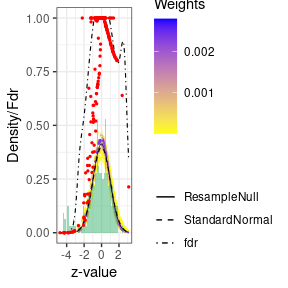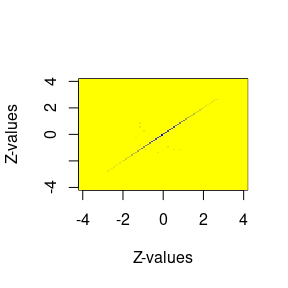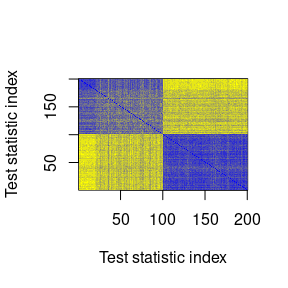# Introduction

The aim of this pacakage is to improve simultaneous inference for correlated hypotheses using collapsed null distributions. These collapsed null distributions are estimated in an empirical Bayes framework through resampling. Wilcoxon rank sum test and two sample t-test are natively implemented, but any other test can be used.

# Installation

library(BiocManager)
BiocManager::install("reconsi")
library(devtools)
install_github("CenterForStatistics-UGent/reconsi")
suppressPackageStartupMessages(library(reconsi))
cat("reconsi package version", as.character(packageVersion("reconsi")), "\n")
## reconsi package version 1.14.0

## General use

We illustrate the general use of the package on a synthetic dataset. The default Wilcoxon rank-sum test is used.

#Create some synthetic data with 90% true null hypothesis
p = 200; n = 50
x = rep(c(0,1), each = n/2)
mat = cbind(
matrix(rnorm(n*p/10, mean = 5+x),n,p/10), #DA
matrix(rnorm(n*p*9/10, mean = 5),n,p*9/10) #Non DA
)
#Provide just the matrix and grouping factor, and test using the collapsed null
fdrRes = reconsi(mat, x)
#The estimated tail-area false discovery rates.
estFdr = fdrRes$Fdr The method provides an estimate of the proportion of true null hypothesis, which is close to the true 90%. fdrRes$p0
##  0.9024115

The result of the procedure can be represented graphically as follows:

plotNull(fdrRes)
## Warning: The dot-dot notation (..density..) was deprecated in ggplot2 3.4.0.
## ℹ Please use after_stat(density) instead.
## ℹ The deprecated feature was likely used in the reconsi package.
##   Please report the issue at
##   <https://github.com/CenterForStatistics-UGent/reconsi/issues>.
## This warning is displayed once every 8 hours.
## Call lifecycle::last_lifecycle_warnings() to see where this warning was
## generated.
## Warning: Removed 2 rows containing missing values (geom_bar()).
## Warning: The guide argument in scale_*() cannot be FALSE. This was deprecated in
## ggplot2 3.3.4.
## ℹ The deprecated feature was likely used in the reconsi package.
##   Please report the issue at
##   <https://github.com/CenterForStatistics-UGent/reconsi/issues>.
## This warning is displayed once every 8 hours.
## Call lifecycle::last_lifecycle_warnings() to see where this warning was
## generated.The approximate correlation matrix of test statistic in the univariate $$h$$ distribution can be visualized as follows:

plotApproxCovar(fdrRes)On the other hand, one can also view the full variance-covariance matrix of the test statistics as found by the resamples. Here we demonstrate how it can recover a block correlation structure in the data.

matBlock = mat
matBlock[x==0,] = matBlock[x==0,] + rep(c(-1,2), each = n*p/4)
fdrResBlock = reconsi(matBlock, x)
plotCovar(fdrResBlock)It is also possible to provide a custom test function, which must accept at least a ‘y’ response variable and a ‘x’ grouping factor. Additionally, quantile, distribution and density functions should be supplied for transformation through quantiles to z-values.

 #With a custom function, here linera regression
fdrResLm = reconsi(mat, x, B = 5e1,
test = function(x, y){
fit = lm(y~x)
c(summary(fit)$coef["x","t value"], fit$df.residual)},
distFun = function(q){pt(q = q, df = q)})

This framework also accepts more than 2 groups, and additional covariates through the “argList” argument.

 #3 groups
p = 100; n = 60
x = rep(c(0,1,2), each = n/3)
mu0 = 5
mat = cbind(
matrix(rnorm(n*p/10, mean = mu0+x),n,p/10), #DA
matrix(rnorm(n*p*9/10, mean = mu0),n,p*9/10) #Non DA
)
#Provide an additional covariate through the 'argList' argument
z = rpois(n , lambda = 2)
fdrResLmZ = reconsi(mat, x, B = 5e1,
test = function(x, y, z){
fit = lm(y~x+z)
c(summary(fit)$coef["x","t value"], fit$df.residual)},
distFun = function(q){pt(q = q, df = q)},
argList = list(z = z))

If the null distribution of the test statistic is not known, it is also possbile to execute the procedure on the scale of the original test statistics, rather than z-values by setting zValues = FALSE. This may be numerically less stable.

fdrResKruskal = reconsi(mat, x, B = 5e1,
test = function(x, y){kruskal.test(y~x)$statistic}, zValues = FALSE) Alternatively, the same resampling instances may be used to determine the marginal null distributions as to estimate the collapsed null distribution, by setting the “resamZvals” flag to true. fdrResKruskalPerm = reconsi(mat, x, B = 5e1, test = function(x, y){ kruskal.test(y~x)$statistic}, resamZvals = TRUE)

When no grouping variable is available, one can perform a bootstrap as resampling procedure. This is achieved by simply not supplying a grouping variable “x”. Here we perform a one sample Wilcoxon rank sum test for equality of the means to 0.

fdrResBootstrap = reconsi(Y = mat, B = 5e1, test = function(y, x, mu){
testRes = t.test(y, mu = mu)
c(testRes$statistic, testRes$parameter)}, argList = list(mu = mu0),
distFun = function(q){pt(q = q,
df = q)})

## Case study

We illustrate the package using an application from microbiology. The species composition of a community of microorganisms can be determined through sequencing. However, this only yields compositional information, and knowledge of the population size can be acquired by cell counting through flow cytometry. Next, the obtained species compositions can multiplied by the total population size to yield approximate absolute cell counts per species. Evidently, this introduces strong correlation between the tests due to the common factor. In other words: random noise in the estimation of the total cell counts will affect all hypotheses. Therefore, we employ resampling to estimate the collapsed null distribution, that will account for this dependence.

The dataset used is taken from Vandeputte et al., 2017 (see manuscript), a study on gut microbiome in healthy and Crohn’s disease patients. The test looks for differences in absolute abundance between healthy and diseased patients. It relies on the phyloseq package, which is the preferred way to interact with our machinery for microbiome data.

#The grouping and flow cytometry variables are present in the phyloseq object, they only need to be called by their name.
data("VandeputteData")
testVanDePutte = testDAA(Vandeputte, groupName = "Health.status", FCname = "absCountFrozen", B = 1e2L)

The estimated tail-area false discovery rates can then simply be extracted as

FdrVDP = testVanDePutte\$Fdr
quantile(FdrVDP)
##           0%          25%          50%          75%         100%
## 1.288044e-19 1.611945e-03 4.919326e-01 1.000000e+00 1.000000e+00

# Session info

Finally all info on R and package version is shown

sessionInfo()
## R version 4.3.1 (2023-06-16)
## Platform: x86_64-pc-linux-gnu (64-bit)
## Running under: Ubuntu 22.04.3 LTS
##
## Matrix products: default
## BLAS:   /home/biocbuild/bbs-3.18-bioc/R/lib/libRblas.so
## LAPACK: /usr/lib/x86_64-linux-gnu/lapack/liblapack.so.3.10.0
##
## locale:
##   LC_CTYPE=en_US.UTF-8       LC_NUMERIC=C
##   LC_TIME=en_GB              LC_COLLATE=C
##   LC_MONETARY=en_US.UTF-8    LC_MESSAGES=en_US.UTF-8
##   LC_PAPER=en_US.UTF-8       LC_NAME=C
##  LC_MEASUREMENT=en_US.UTF-8 LC_IDENTIFICATION=C
##
## time zone: America/New_York
## tzcode source: system (glibc)
##
## attached base packages:
##  stats     graphics  grDevices utils     datasets  methods   base
##
## other attached packages:
##  reconsi_1.14.0
##
## loaded via a namespace (and not attached):
##   gtable_0.3.4            xfun_0.40               bslib_0.5.1
##   ggplot2_3.4.4           ks_1.14.1               rhdf5_2.46.0
##   Biobase_2.62.0          lattice_0.22-5          rhdf5filters_1.14.0
##  vctrs_0.6.4             tools_4.3.1             bitops_1.0-7
##  generics_0.1.3          biomformat_1.30.0       stats4_4.3.1
##  parallel_4.3.1          tibble_3.2.1            fansi_1.0.5
##  cluster_2.1.4           pkgconfig_2.0.3         KernSmooth_2.23-22
##  Matrix_1.6-1.1          data.table_1.14.8       S4Vectors_0.40.0
##  lifecycle_1.0.3         GenomeInfoDbData_1.2.11 farver_2.1.1
##  compiler_4.3.1          stringr_1.5.0           Biostrings_2.70.0
##  munsell_0.5.0           codetools_0.2-19        permute_0.9-7
##  GenomeInfoDb_1.38.0     htmltools_0.5.6.1       sass_0.4.7
##  RCurl_1.98-1.12         yaml_2.3.7              pracma_2.4.2
##  pillar_1.9.0            crayon_1.5.2            jquerylib_0.1.4
##  MASS_7.3-60             cachem_1.0.8            vegan_2.6-4
##  iterators_1.0.14        mclust_6.0.0            foreach_1.5.2
##  nlme_3.1-163            tidyselect_1.2.0        digest_0.6.33
##  mvtnorm_1.2-3           stringi_1.7.12          dplyr_1.1.3
##  reshape2_1.4.4          labeling_0.4.3          splines_4.3.1
##  Rhdf5lib_1.24.0         plyr_1.8.9              zlibbioc_1.48.0